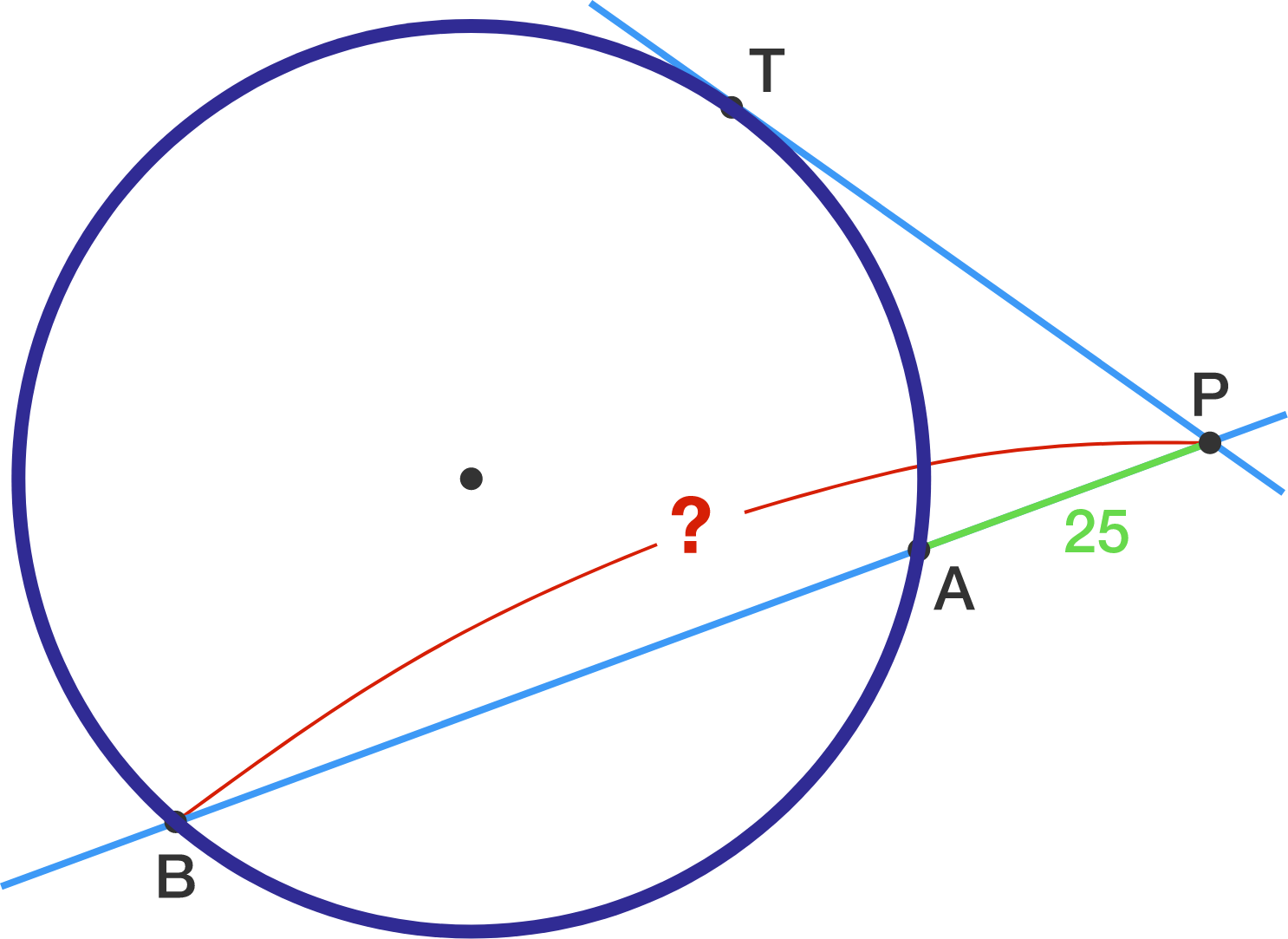# Peculiar Point P

Geometry Level 3

A point $P$ is given outside of a circle $\Gamma$. A tangent from $P$ touches $\Gamma$ at $T$ with $PT=45$. A line from $P$ cuts $\Gamma$ at the 2 points $A, B$. If $PA=25$, what is the length of $PB$?×